# Sample Question: HMO Theory for the Allyl Cation, Radical and Anion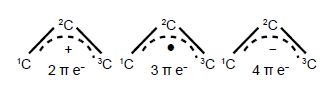=> Write down the Hiickel secular determinant for the acyclic three C-atoms in terms of x, where x = (α – E)/ β.

=> Solve the secular determinant for x and then rewrite the solution in terms of (α –  E)/ β  and so find the three allowed quantized energies (eigenvalues).

=> Evaluate the normalized wavefunction (eigenvector) for each energy (eigenvalue).

=> Which of the wavefunctions is the HOMO, LUMO or SOMO (singly occupied MO) as appropriate, for each of the allyl cation, radical and anion molecules?

Solution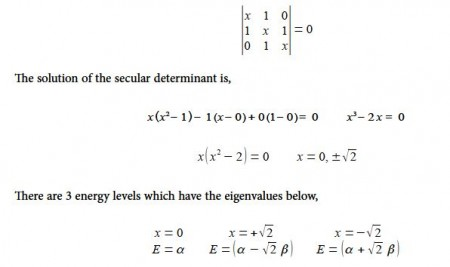Since α and β are both have negative values so with increasing energy the eigenvalues are the following

E1 = α + √2 β

E2 = α

E3 = α – √2 β

To find the three eigenvectors (wavefunctions) corresponding to these three eigenvalues we must separately solve the Bickel matrix for ally’ for each energy For E1 = α + √2 β then (α – E1) = – √2 β.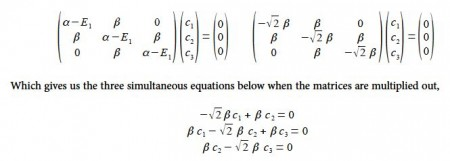Subtracting the third equation from the first gives c1 = c3. From the first equation c2 = √2 c1, and from the third equation c2 = √2 c3 which when we normalize the coefficients using c12 + c22+ c32 = 1 gives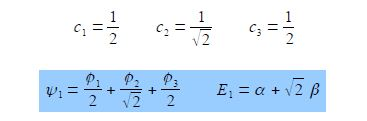From the first and third simultaneous equations c2 = 0, and from second equation, c1 = – c3 If the coefficients are normalized, then c12 + c22 + c32 = 1 then,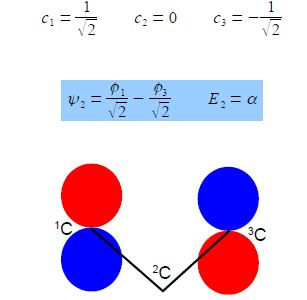Substituting into the Hiickel matrix for ally) with E3 = α – √2 β then (α – E3) = √2 β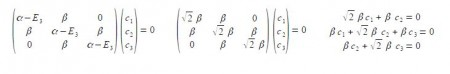Subtracting the third from the first simultaneous equations c1 = c3 from the first equation c2 = √2 c1; from the third equation c2 = – √2 c3 thus when the coefficients are normalised, then c12 + c22 + c32 = 1 we have,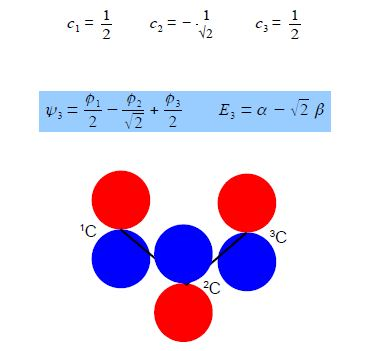Below Figure shows the MO energy diagrams for the three allyl species.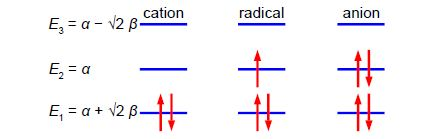In the allyl cation CH2=CH—CH2+ with 2 π-electrons, then ψ1 is the HOMO and ψ2 is the LUMO. In the allyI radical CH2=CH—CH2+ with 3 π-electrons, then ψ1 has 2 π-electrons and ψ2 is the SUMO with 1 π-electron and ψ3 is the LUMO. In the allyl anion CH2=CH—CH2 with 4 π-electrons, then ψ1 has 2 π -electrons and ψ2 is the HOMO with 2 π-electrons and ψ3 is the LUMO.